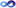검색어 입력폼

# [알고리즘] Knapsack Problem 되추적 기법 구현

등록일 2004.04.22C언어 (cpp) | 2페이지 | 가격 2,000원

## 소개글

Knapsack Problem 되추적 기법 구현

없음

## 본문내용

#include <stdio.h>
#include <stdlib.h>
#include <time.h>

time_t t;
int numbest = 0;
int maxprofit = 0;
int P,W;
double CAPA = 0.0;
bool include,bestset;

bool promising(int i, int profit, int weight, int NUMOFITEMS)
{
int j, k;
int totweight;
int bound;

if (weight >= CAPA)
return false;
else {
j = i + 1;
bound = profit;
totweight = weight;
while (j <= NUMOFITEMS && totweight + W[j] <= CAPA){
totweight += W[j];
bound += P[j];
j++;
}
k = j;
if (k <= NUMOFITEMS)
bound = bound + (CAPA - totweight) * P[k] / W[k];

return (bound > maxprofit);
}
}

없음